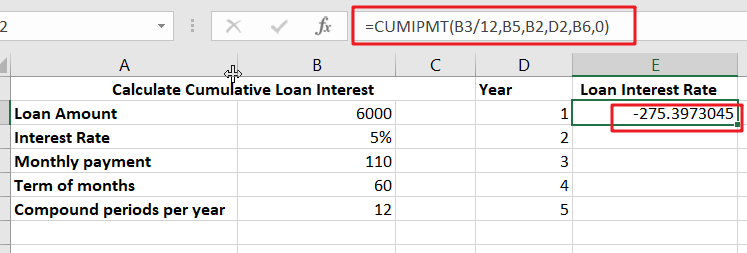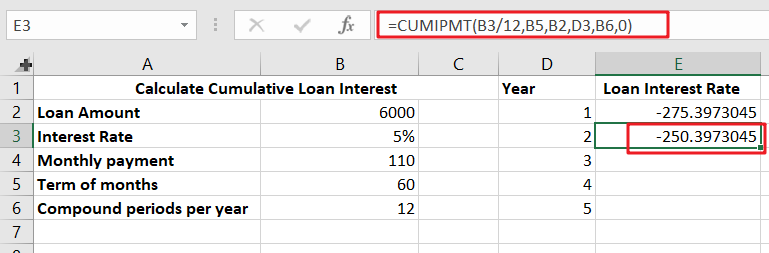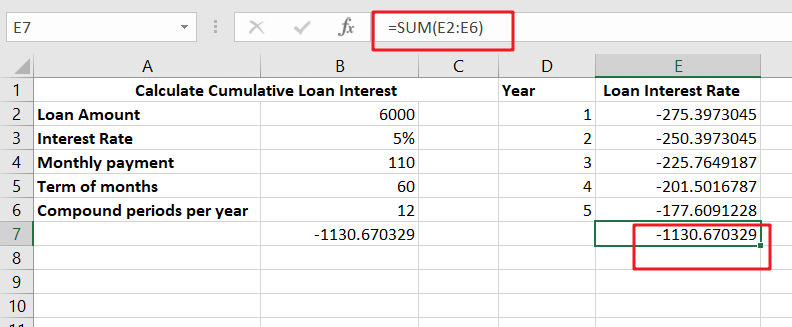# Calculate Loan Interest in Given Year

When you borrow money, you are supposed to repay it gradually. Lenders, on the other hand, want to be compensated for their services and the risk they incur by lending you money. That is, you will not just repay the money you borrowed. You’ll repay the loan plus interest.

Interest is a significant source of revenue for lenders, banks, and credit card issuers. Here’s an overview of how interest works and how to calculate loan interest in given year using Excel CUMIPMT function.## What is interest on a loan?

Interest is the cost of borrowing money from another person. If you borrow \$6,000 for a personal loan, you may end up owing the lender about \$7,130 in interest over the next five years. That additional \$1,130 is interest.As you return the loan over time, a part of each payment is applied to the amount borrowed (referred to as the principal), and the remainder is applied to interest expenses. The amount of loan interest charged by the lender is decided by factors such as your credit history, income, loan amount, loan conditions, and existing debt load.

## Calculate loan interest in given year

To maximize earnings, lenders charge interest in a variety of ways. Calculating loan interest may be challenging, since many forms of interest need additional mathematics.

## Simple interest

Provided a lender employs the simple interest technique, calculating loan interest is straightforward if you have the necessary information. Collect information such as the principal loan amount, the interest rate, and the total number of months or years you intend to repay the loan.

## Calculation

You may use the following calculation to get your total interest: Interest is calculated as the principal loan amount multiplied by the interest rate multiplied by the time period (a.k.a. the number of years in the term).

For instance, if you get a five-year loan for \$20,000 at a rate of 5%, the basic interest calculation is as follows:

20,000 multiplied by .05 multiplied by five equals \$5,000 in interest.

On short-term loans, you may find basic interest. However, the manner in which the majority of banks and lenders calculate interest is more sophisticated.

## Amortizing loans

Numerous lenders assess interest on an amortization schedule. Student loans, mortgages, and automobile loans are often included in this category. While the monthly payment on these loans is set and the loan is paid in equal installments over time, the lender’s method of applying your payments to the loan amount varies over time.

With amortizing loans, the early payments are often high in interest, which means that less of the money you spend each month goes toward paying down the principal loan balance.

However, as time passes and you approach the payback date of your loan, the tables flip. Toward the conclusion of the term of your loan, the lender will apply the bulk of your monthly payments to the principal debt and less to interest costs.

## Calculation

Divide your interest rate by the total number of payments that year. If your interest rate is 6% and you make monthly payments, divide 0.06 by 12 to get 0.005.

Multiply that figure by the remaining loan total to get the amount of interest you’ll pay that month. If your loan sum is \$5,000, your first month’s interest will be \$25.

Subtract the interest from your set monthly payment to get the amount of principal due in the first month. If your lender has said that your fixed monthly payment would be \$430.33, you will make the first month’s payment of \$405.33 toward the principal. This sum is deducted from your current balance.

Repeat the procedure the next month with your new remaining loan amount, and continue doing so for each future month.